# Spherical harmonics, method of

A method for obtaining an approximate solution of a kinetic equation by the decomposition of the phase density of the particles into a finite sum of spherical functions in arguments that define the direction of the velocity of the particles (see ). The method is widely used in the solution of problems of neutron physics.

In one-dimensional plane geometry the stationary, integro-differential, kinetic transport equation (given an isotropic scattering of the particles)(1)

is replaced by an approximate system of differential equations for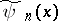— the approximate values of the Fourier coefficients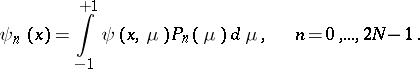(2)

A system of the form(3)

arises, under the condition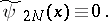(4)

Here,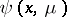is the phase density of the particles that are scattered in the matter,is the average number of secondary particles arising from one act of interaction with the particles of matter, and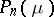is the Legendre polynomial of degree(cf. Legendre polynomials). System (3) defines the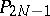-approximation of the method of spherical harmonics for equation (1). The approximate value of the phase density is(5)

For equation (1), the typical boundary conditions take the form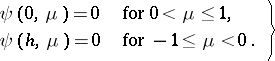(6)

Boundary conditions of this type exist in, for example, the problem in neutron physics of the critical conditions of a layer of thicknesswith free surfacesand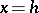(boundaries with vacuum). In this problem, a positive solution of (1) and (6), as well as the eigenvalue, must be found.

In the method of spherical harmonics, it is natural to replace (6) by(7)

However, this approach involves twice as many conditions than are required for a partial solution of (3). In practice, a different set of values has been tested in (7). The best result is obtained by conditions with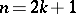,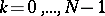. For the single-velocity transport equation of a general type, the Vladimirov variational principle leads to a system of equations of the method of spherical harmonics and the given boundary conditions (when choosing the experimental functions in the form of a linear combination of spherical harmonics). In three-dimensional geometry, the boundary conditions can be written in the form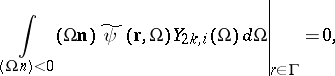(8)Hereis a vector of space coordinates,is a unit vector of the velocity of the particle with spherical coordinates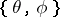,is the unit vector of the exterior normal to the piecewise-smooth surfacethat bounds the convex domain in space in which the problem is solved,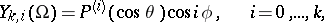are the spherical functions,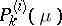are the associated Legendre functions of the first kind (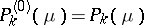are the Legendre polynomials).

The lowest approximationsof the method of spherical harmonics are widely used in solving problems of neutron physics and give good results well away from the boundaries of the domain, sources and strong neutron absorbers. The theory of growth is also constructed in a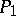-approximation. The generalized solution of the method of spherical harmonics converges to the solution of the transport equation when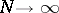(see ). The rate of the convergence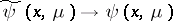is easily estimated, by comparing the integral equations for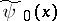and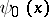, i.e. by estimating the proximity of their kernels. Equation (1) with the boundary conditions (6) reduces to an integral equation with the kernel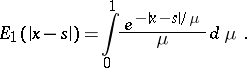System (3), given boundary conditions analogous to (6),(9)

where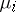are the roots of, reduces to an integral equation with kernel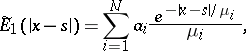where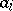are the weights of the Gauss quadrature formula for the system of nodes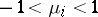. The singularity of the functionleads to a slow convergence for large: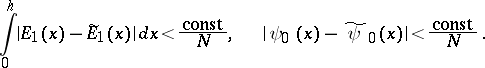The approximate eigenvalue converges to an exact eigenvalue at a rate of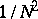.

The boundary conditions (9) arise naturally in the solution of the kinetic equation by the method of discrete ordinates, which consists of replacing (1) by the approximate system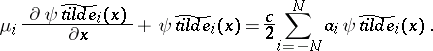(10)

The method of discrete ordinates in one-dimensional geometry is equivalent to the method of spherical harmonics (see ), since the system (10) can be obtained from (3) by means of a linear transformation of the unknown functions: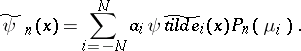However, in multi-dimensional problems, the method of spherical harmonics in the lowest approximations is more accurate than the method of discrete ordinates.